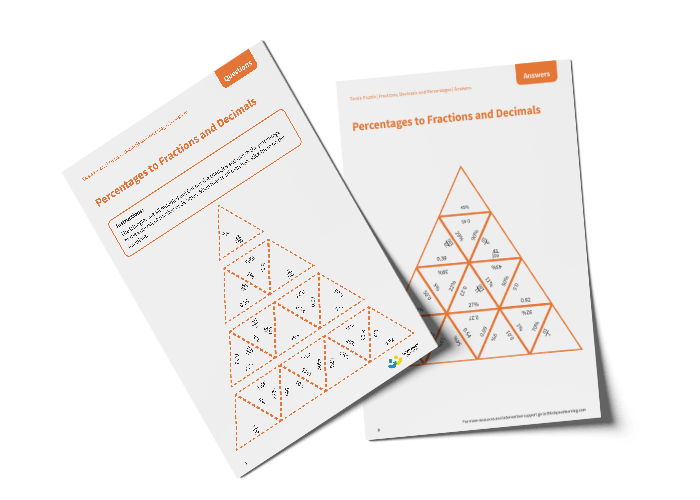# Tarsia Puzzle Percentages to Fractions and Decimals (Year 5)

The triangles in the tarsia puzzle have all been mixed up. In this converting percentages to fractions and decimals tarsia puzzle, pupils will need to match the percentage to the equivalent fraction or decimal to create one large triangle.

This Year 5 percentages to fractions and decimals tarsia puzzle worksheet contains:

• One question sheet
• One template sheet

Year 5

Fractions, Decimals, Percentages